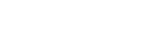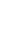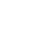# Shear Strength of Soil by Direct Shear Test

Direct shear test or Box shear test is used to determine the shear strength of the soil. It is more suitable for cohesionless soils.

## Shear Strength of Soil by Direct Shear Test

Shear strength of a soil is its maximum resistance to shearing stresses. The shear strength is expressed asWhere   C’ = Effective cohesion= Effective stress= Effective angle of shearing resistance

Using direct shear test, one can find out the cohesion and angle of internal friction of soil which are useful in many engineering designs such as foundations, retaining walls, etc.

This test can be performed in three different drainage conditions namely unconsolidated-undrained, consolidated-undrained and consolidated-drained conditions. In general, cohesionless soils are tested for direct shear in consolidated drained condition.

## Apparatus Required for Direct Shear Test

Apparatus required for conduction direct shear test are

• Shear box
• Shear box container
• Base plate with cross groves on its top
• Porous stones (2 Nos)
• Plain Grid plates (2Nos)
• Perforated grid plates (2Nos)
• Digital weighing machine
• Proving ring
• Dial gauges (2 Nos)
• Weights
• Tampering Rod
• Spatula
• Rammer
• SamplerFig 1: Direct Shear Test Apparatus

## Test Procedure of Direct Shear Test

Test Procedure of Direct shear test contains following steps:

1. Collect the soil specimen which is either undisturbed or remolded. The sample should be taken using sampler and Rammer. If cohesionless soil is taking then sampler and rammer are not required.
2. The inner dimensions of sampler should be 60 mm x 60 mm in plan which are also the inner dimensions of shear box. The Thickness of box is about 50 mm while the thickness of sample should be 25mm.3. Now attach the two halves of the shear box with locking pins and place the base plate at the bottom.
4. Above the bottom plate, Place the porous stone and above it place the grid plate. Plain grid plates are used for undrained conditions while perforated grid plates are used for drained conditions.
5. Now we have baseplate, porous stone and grid plate in the shear box. Weigh the box at this stage and note down.
6. After that place the soil specimen above the grid plate. Undisturbed sample is directly transferred to shear box. If sandy soil is using, place it layers wise and tamper the each layer to get the required density.
7. Note down the weight of shear box with soil specimen.
8. Above the soil specimen, place the upper grid plate, porous stone and loading pad one above the other.Fig 3: Different Layers Positions in Shear Box

9. Now the whole box is placed in a container and mounted on the loading frame.
10. Proving ring is arranged in such a way that it should contact the upper half of the shear box.
12. Two dial gauges are fitted one to the container for measuring shear displacement and other one is to the loading yoke for measuring vertical displacement.
13. Now locking pins are removed from the shear box and spacing screws are placed in their respective positions of the box.Fig 4: Applying Load on Specimen

14. The upper half of the box is raised slightly with the help of spacing screws. The spacing is decided depending upon the maximum size of particle.
15. Now apply the normal stress which is generally 25 kN/m2. Also apply the shear load at a constant rate of strain.
16. Now the box starts reacting to loads applied and for every 30 seconds note down the readings of proving ring and dial gauges.
17. If the proving ring reaches maximum and suddenly drops it, means the specimen is failed. Note down the maximum value which is nothing but failure stress.
18. For some soils, failure point is taken at 20% of shear strain.
19. Finally remove the box and measure the water content of the specimen.
20. Repeat the same procedure for different normal stresses of 50, 100, 150,200,250,300,400 kN/m2

## Observations and Calculations for Direct Shear Test

Calculations are to be done using the observations taken from the test as follows:

• Size of the box =
• Area of the box =
• Thickness of specimen =
• Mass of specimen =
• Volume of specimen =
• Bulk Density of soil =
• Dry density of soil =
• Void ratio =
• Mass of box + base plate + porous stone + grid plate =
• Mass of box + base plate + porous stone + grid plate + Soil specimen =

Calculate the shear stress using below tabulated observations:

 S.No Elapsed time Horizontal dial gauge reading Horizontal displacement Vertical dial gauge reading Vertical displacement Proving ring reading Shear force Shear stress

Now determine the shear stress at failure for different normal stress values:

 Test no. Normal stress (kN/m2) Shear Stress at Failure (kN/m2) Shear Displacement at Failure Initial Water Content Final Water Content

Now plot a graph between normal stress and shear stress by taking normal stress on abscissa and shear stress at failure on ordinate. The graph looks like as shown below.Fig 5: Graph plotted between Normal Stress and Shear stress

From the graph cohesion intercept (c’) and angle of shearing resistance () can be known and shear strength (s) can be calculated from the formula.## Result of Direct Shear Test

Shear strength of the given soil sample is = ______________ kN/m2.